# Scatter plots in R Language

A scatter plot is a set of dotted points representing individual data pieces on the horizontal and vertical axis. In a graph in which the values of two variables are plotted along the X-axis and Y-axis, the pattern of the resulting points reveals a correlation between them.

## R – Scatter plots

We can create a scatter plot in R Programming Language using the plot() function.

Syntax: plot(x, y, main, xlab, ylab, xlim, ylim, axes)

Parameters:

• x: This parameter sets the horizontal coordinates.
• y: This parameter sets the vertical coordinates.
• xlab: This parameter is the label for horizontal axis.
• ylab: This parameter is the label for vertical axis.
• main: This parameter main is the title of the chart.
• xlim: This parameter is used for plotting values of x.
• ylim: This parameter is used for plotting values of y.
• axes: This parameter indicates whether both axes should be drawn on the plot.

## Simple Scatterplot Chart

In order to create Scatterplot Chart:

1. We use the data set “mtcars”.
2. Use the columns “wt” and “mpg” in mtcars.

Example:

## R

 `input <- mtcars[, ``c``(``'wt'``, ``'mpg'``)]` `print``(``head``(input))`

Output: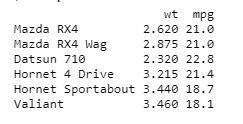## Creating a Scatterplot Graph

In order to create an R Scatterplot graph:

1. We are using the required parameters to plot the graph.
2. In this ‘xlab’ describes the X-axis and ‘ylab’ describes the Y-axis.

Example:

## R

 `# Get the input values.` `input <- mtcars[, ``c``(``'wt'``, ``'mpg'``)]`   `# Plot the chart for cars with` `# weight between 1.5 to 4 and` `# mileage between 10 and 25.` `plot``(x = input\$wt, y = input\$mpg,` `    ``xlab = ``"Weight"``,` `    ``ylab = ``"Milage"``,` `    ``xlim = ``c``(1.5, 4),` `    ``ylim = ``c``(10, 25),        ` `    ``main = ``"Weight vs Milage"` `)`

Output: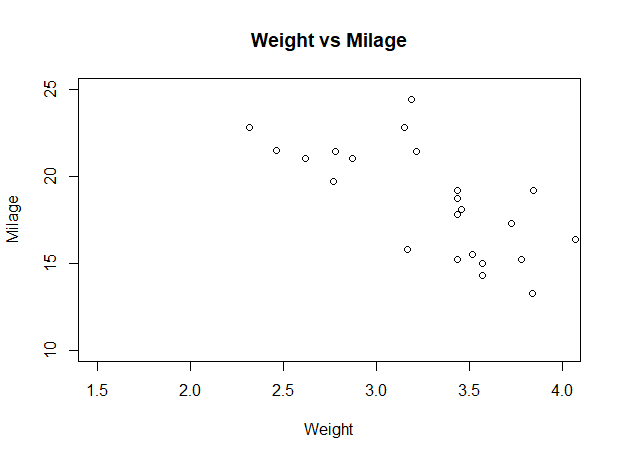Scatter plots in R Language

## Scatterplot Matrices

When we have two or more variables and we want to correlate between one variable and others so we use a R scatterplot matrix.

pairs() function is used to createR  matrices of scatterplots.

Syntax: pairs(formula, data)

Parameters:

• formula: This parameter represents the series of variables used in pairs.
• data: This parameter represents the data set from which the variables will be taken.

Example:

## R

 `# Plot the matrices between` `# 4 variables giving 12 plots.`   `# One variable with 3 others` `# and total 4 variables.` `pairs``(~wt + mpg + disp + cyl, data = mtcars,` `    ``main = ``"Scatterplot Matrix"``)`

Output: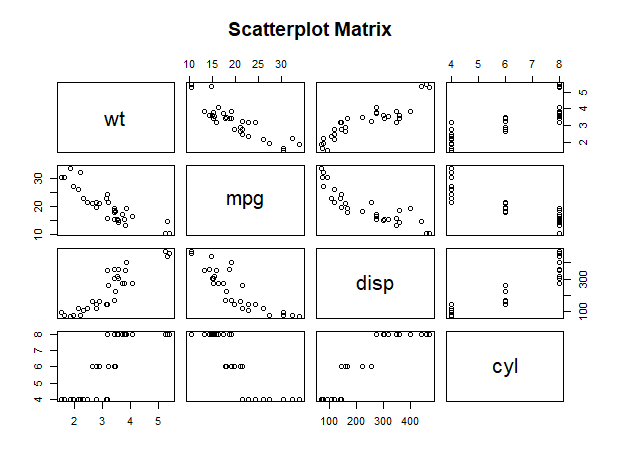Scatter plots in R Language

## Scatterplot with fitted values

In order to create R Scatterplot Chart:

1. We are using the ggplot2 package provides ggplot() and geom_point() function for creating a scatterplot.
2. Also we are using the columns “wt” and “mpg” in mtcars.

Example:

## R

 `# Loading ggplot2 package` `library``(ggplot2)` `    `  `# Creating scatterplot with fitted values.` `# An additional function stst_smooth` `# is used for linear regression.` `ggplot``(mtcars, ``aes``(x = ``log``(mpg), y = ``log``(drat))) +` `        ``geom_point``(``aes``(color = ``factor``(gear))) +` `        ``stat_smooth``(method = ``"lm"``,` `        ``col = ``"#C42126"``, se = ``FALSE``, size = 1` `)`

Output: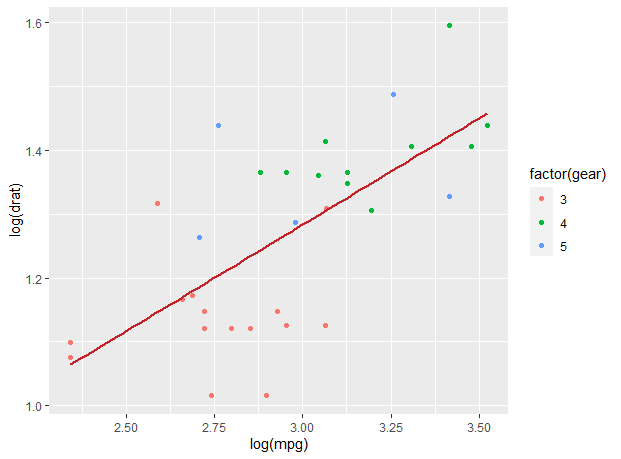Scatter plots in R Language

## Adding title with dynamic name

To create R Scatterplot Chart, Add a sub-title:

1. We use the additional function, In ggplot we add the data set “mtcars” with this adding ‘aes’, ‘geom_point’.
2. Use the Title, Caption, Subtitle.

Example:

## R

 `# Loading ggplot2 package` `library``(ggplot2)` `    `  `# Creating scatterplot with fitted values.` `# An additional function stst_smooth` `# is used for linear regression.` `new_graph<-``ggplot``(mtcars, ``aes``(x = ``log``(mpg),` `                              ``y = ``log``(drat))) +` `                    ``geom_point``(``aes``(color = ``factor``(gear))) +` `                    ``stat_smooth``(method = ``"lm"``,` `                                ``col = ``"#C42126"``,` `                    ``se = ``FALSE``, size = 1)`   `# in above example lm is used for linear regression` `# and se stands for standard error.` `# Adding title with dynamic name` `new_graph + ``labs``(` `        ``title = ``"Relation between Mile per hours and drat"``,` `        ``subtitle = ``"Relationship break down by gear class"``,` `        ``caption = ``"Authors own computation"` `)`

Output: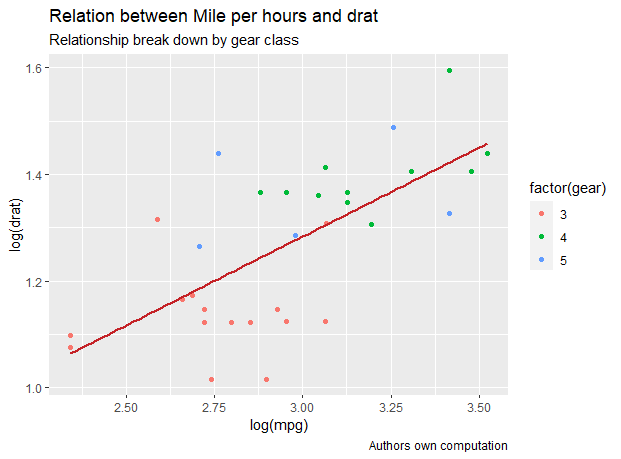Scatter plots in R Language

## 3D Scatterplots

Here we will use R scatterplot3D package to create 3D scatterplots, this package can plot R scatterplots in 3D using scatterplot3d() methods.

## R

 `# 3D Scatterplot` `library``(plotly)` `attach``(mtcars)` `plot_ly``(data=mtcars,x=~mpg,y=~hp,z=~cyl,color=~gear)`

Output: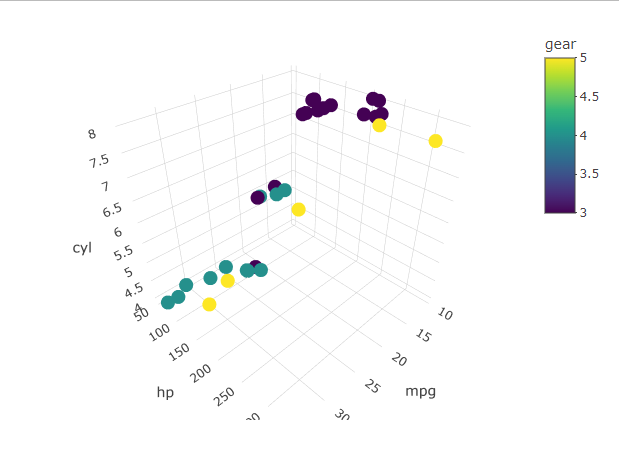Scatter plots in R Language

Whether you're preparing for your first job interview or aiming to upskill in this ever-evolving tech landscape, GeeksforGeeks Courses are your key to success. We provide top-quality content at affordable prices, all geared towards accelerating your growth in a time-bound manner. Join the millions we've already empowered, and we're here to do the same for you. Don't miss out - check it out now!

Previous
Next